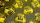# PIN - codes

How many five-digit PIN - code can we create using the even numbers?

Result

x =  3125

#### Solution:Leave us a comment of example and its solution (i.e. if it is still somewhat unclear...):

Showing 0 comments:Be the first to comment!#### To solve this verbal math problem are needed these knowledge from mathematics:

Would you like to compute count of combinations?

## Next similar examples:

1. Weekly serviceIn the class are 20 pupils. How many opportunities have the teacher if he wants choose two pupils randomly who will weeklies?
2. MenuOn the menu are 12 kinds of meal. How many ways can we choose four different meals into the daily menu?
3. Olympics metalsIn how many ways can be win six athletes medal positions in the Olympics? Metal color matters.
4. MedalsIn how many ways can be divided gold, silver and bronze medal among 21 contestant?
5. ConfectioneryThe village markets have 5 kinds of sweets, one weighs 31 grams. How many different ways a customer can buy 1.519 kg sweets.
6. ChordsHow many 4-tones chords (chord = at the same time sounding different tones) is possible to play within 7 tones?
7. Three workshopsThere are 2743 people working in three workshops. In the second workshop works 140 people more than in the first and in third works 4.2 times more than the second one. How many people work in each workshop?
8. ProbabilityWhat are the chances that the lottery, in which the numbers are drawn 5 of 50 you win the first prize?
9. Count of trianglesGiven a square ABCD and on each side 8 internal points. Determine the number of triangles with vertices at these points.
10. VariationsDetermine the number of items when the count of variations of fourth class without repeating is 42 times larger than the count of variations of third class without repetition.
11. Theorem proveWe want to prove the sentence: If the natural number n is divisible by six, then n is divisible by three. From what assumption we started?
12. Elimination methodSolve system of linear equations by elimination method: 5/2x + 3/5y= 4/15 1/2x + 2/5y= 2/15
13. SequenceWrite the first 6 members of these sequence: a1 = 5 a2 = 7 an+2 = an+1 +2 an
14. TrigonometryIs true equality? ?
15. LineIt is true that the lines that do not intersect are parallel?
16. Sequence 2Write the first 5 members of an arithmetic sequence a11=-14, d=-1
17. SequenceWrite the first 7 members of an arithmetic sequence: a1=-3, d=6.